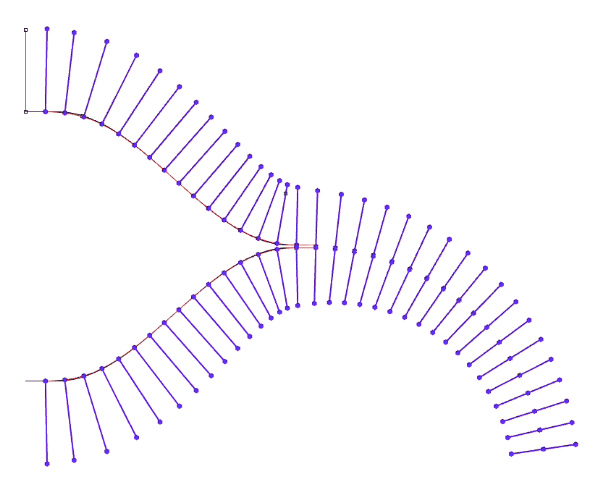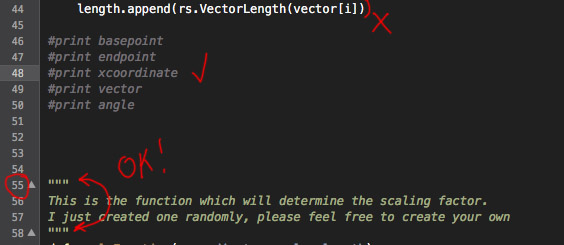# Batch Scale Curves

Hello everyone.
I’m wanting to scale all of the curves individually. I’ve been going through the Designalyze, Mode Lab, Jose Sanchez and Parametric House tutorials, but being the monkey-see-monkey-do type haven’t seen an example of scaling I can copy and fit to my purpose in any of them yet.
Each (straight) curve has it’s own factor to scale by which is a function of it’s base point x-coordinate and angle relative to the z-axis. I’ve started a script to try and get moving on it but hit the wall when it came to the matrix parameter. Looking at the TransformObjects help, I’m not sure how to proceed. I’ve scoured the forum for “scale” and the posts are encouraging, but still a mystery.
Any help is appreciated.
Thanks,
David

Batch_Scale_Curves.3dm (86.3 KB)
Batch_Scale_Curves.py (758 Bytes)

Hello @saucerdesigner,
`rs.ScaleObjects` can be used to scale objects. Is that what you need?

Hey,

I am attaching a grasshopper file with the code in a GHPython component.
It should do what you need (if I understood correctly).
I hope this helps.

scaling.gh (3.7 KB)

Thank you @pragyagupta171.

I cleaned up the 3dm file to show only the curves I wanted to scale.
When I opened the file in Grasshopper, I received this Grasshopper Event Log: (see attached)
The latest version of GH for for my version of Rhino 5 seems to be 0.9.0076. I ran it anyway, set Multiple Curves by selecting all, set the scale factor to 1.5, and it seemed to have no effect.

I sincerely appreciate your taking the time to help.

David

Thank you @Dancergraham

That just might do it! Thanks!

I’m assuming compatibility is the issue. The easiest thing would be to copy the code into your own file. I tried the code and it works perfectly. So a simple copy and paste should work!

Also, where did you set the scale factor?

That (copying the components from your .gh into a blank canvas of my version) was the first thing I tried after attempting to run it as it was. The result was the same. I’ll try using components from my version if I can figure out how to extract the script from your GHPython script component.

I entered the scale factor 1.5 by usijg Set Number in the Unit Z vector component.

The curves on the top of the sinusoidal segment, once scaled, could be mirrored across the x-axis.
The curves on the top and bottom of the circular arc segment, however have to be scaled separately.

The pseudo-code for a Python script might go something like this:

``````create a list of all the curves to be scaled
for each curve in the list of curves to be scaled:
get the x-coordinate of the start (the end of the curve nearest the baseline)
of the existing curve
get the angle betwee the curve and vertical
from the formula having the x-coordinate and angle from vertical as
variables, apply the unique scale factor
end
``````

Double click on the python component, it opens the code editor. The z vector is only being used to find the angle relative to z and not for scaling. The scaling factor is within the code.

Is something like this what you are looking for?You can determine the size of the lines by minimum and maximum values.

Batch_Scale_Curves2.gh (17.4 KB)

I have re-created the definition using components from my version. I copied your script into my version of the GHPython script component and deleted a duplicate curve in the 3dm file. I still get nothing but the following in the message balloon attached to the script component: 1. Solution exception:iteration over non-sequence of type Guid. My compliments to you on your understanding of what I am trying to do.

Thanks,
David

Batch_Scale_Curves_06_29_19.3dm (92.3 KB)
my_scaling.gh (8.5 KB)

Hello @diff-arch,

Thank you. I’ll check it out.

David

You need to give list access and not item access

1 Like

Wow!Thank you, @pragyagupta171

Now I’ll work on plugging my scaling function into the script. By the way, the formula I use does not give me a scale factor, but rather a desired length. If I can get the length of the curve to be scaled I can use it as the devisor of the desired length to obtain the scale factor.
Here’s the formula,
where:
L = the desired length of the scaled curve
r = xcoordinate
ɑ = angle from vertical
A= area of the conical frustum = 40.9 [sq. in.]

Thank you, @diff-arch

The objective is to scale each existing curve so that it represents the slant height of a conical frustum having an area of 40.904 [sq. in].

The formula for finding the slant height, which is what the curves will be scaled to is in the form of a quadratic equation:

scale_factor = ((xcoordinate*-1)+(math.sqrt((((math.pow(xcoordinate,2))+(40.904/math.pi)*math.sin(angle))))))/length

where:
xcoordinate = starting point of the existing curve
angle = angle in degrees from vertical
length = length of the existing curve

I had @pragyagupta171’s suggestion working fairly well for a while but something changed and I haven’t figured out what yet. My working version wasn’t saved so I tried to reconstruct it but it’s giving me syntax errors that are driving me nuts.

my_scaling_06_29_19.gh (15.5 KB) .

Batch_Scale_Curves.3dm (50.3 KB)

I hope this helps.

David

The GHPython component tells you what and where the errors occur, you just have to look at the debugging information and inspect your code in the right locations.

On line 55, as already mentioned by you, you have a syntax error. If you look closely at line 55, you notice that the code description is correctly commented out with a pair of triple quotes, so you have to inspect the code above line 55. On lines 50 to 46, all your print statements are also correctly commented out, however on line 44, `length.append(rs.VectorLength(vector[i]))` is missing the closing parenthesis.After correcting this, you get a value conversion error. The component tells you that on line 42, it failed to convert the `Rhino.Geometry.Vector3d` object to a Vector3d.
At first, this might sound weird, right, but when you `print type(vector[i])` and `print type(y)`, you’ll notice that `vector[i]` is indeed a Vector3d, but `y` - your component input that gets fed in the unit z-vector -, is in fact a list (not a vector). Computing the vector angle between a vector and a list is impossible! What you need to do here is, change your component input y to Item Access. There is no need to input single items as lists. If you input a vector as a list, it gets converted to a list of length 3 with the former vector coordinates as list items.

The script seems to work fine after that.

1 Like

Here’s an updated, cleaned up version of @pragyagupta171’s script:

``````"""Provides a scripting component.
Inputs:
x: Directional curves (List Access)
y: World z-axis (Item Access)
Output:
a: The scaled curves"""

__author__ = "p1r4t3b0y, pragyagupta171"
__version__ = "2019.06.30"

import rhinoscriptsyntax as rs
import math

# Functions/methods get declared above 'free' code
def get_scale_factor(x, angle, length, area=40.904):
"""Returns the scale factor."""
f = ((x * -1) + (math.sqrt((((x**2) + (area / math.pi) \
* math.sin(angle)))))) / length
return f

scaled_curves = [] # list of scaled curves

for i in range(len(x)):
# Loop every index from 0 to the list length - 1 of all curves (x)
curr_curve = x[i] # current curve

# Get the start/base point of the current curve
base_point = rs.CurveStartPoint(curr_curve)

# Get the tangential vector of the current curve
curve_vec = rs.VectorCreate(end_point, base_point)

# Get the angle between the curve vector and the world z-axis
angle = rs.VectorAngle(crv_vec, y)

# Get the scale factor of the current curve
scale_factor = get_scale_factor(base_point.X, angle, crv_vec.Length)
print scale_factor

# Create the scale transformation, scale and store the new curve
xform = rs.XformScale(scale_factor, base_point)
scaled_curve = rs.TransformObject(curr_curve, xform, True)
scaled_curves.append(scaled_curve)

a = scaled_curves
``````

You don’t need to store every single variable/parameter in lists, and loop numerous times over the same initial curves list. It can all be done in a single for loop!
The logic behind this is pretty simple. What you try to achieve concerns every curve individually, so you loop all the curves and evaluate what needs evaluating at each iteration for the current curve. The result of the evaluation at each iteration, the scaled curve, is then saved outside the loop in a list of scaled curves, which is finally output through component output a.

Hey, everyone! I want to express my profound and sincere appreciation to each of you and to all of the Rhino community for your generous and amazing support.

I decided to try implementing my scaling function into pragyagupta171’s script again and by re-typing my scaling algorithm placing parenthesis differently it works beautifully.

Thank you!Thank you!Thank you!

David

my_scaling_06_30_19.gh (12.1 KB)

4 Likes

Hello again, everyone.
@pragyagupta171, @Dancergraham, @diff-arch
Well, it works, but not as beautifully as I thought. When I baked the scaled curves and created a curve through the endpoints using Interpolate Curve, what I got was disappointing.
Control Points was not satisfactory either. I recall seeing something, by Dale Fugier, I believe, about the limitations of the Transformation function in GH.

Is what I’m seeing what he was talking about?
I applied the Transform>Smooth option for a dozen or so times (x and z) and it didn’t seem to help that much.

Any ideas?

Thanks,

David

Edit: =========================================================================
As is often the case, @pascal sorted it out. The problem was my not having converted the angles to Radians. Voila!

my_scaling_06_30_19.gh.3dm (108.4 KB)

my_scaling_06_30_19.gh (13.6 KB)